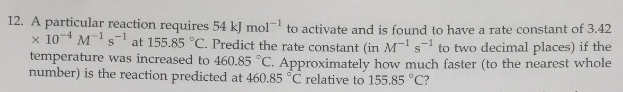# 12. A particular reaction requires 54 kJ mol^-1 to activate and is found to have a rate constant of 3.42 x 10^-4 M^-1 s ^-1 at 155.85 degrees C. Predict the rate constant (in M^-1 s^-1 to two decimal places) if the temperature was increased to 460.85 degrees C. Approximately how much faster (to the nearest whole number) is the reaction predicted at 460.85 degrees C relative to 155.85 degrees C?